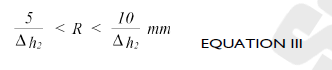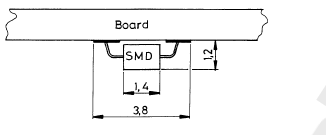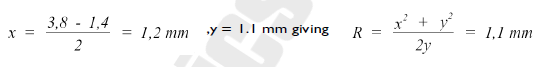# Calculations on the Relation between Solderwave Pressure and Solder Penetration in Gaps

Introduction
For the soldering of SMD's mounted on the solder side of a printed circuit board, the wave pressure to the board must in general be higher than for soldering components with protruding leads on the solder side. With higher wave pressures the risk of solder flooding via gaps in the board increases. In this article the relation between solder pressure, gap dimensions and wetting power will be explained, using a calculation model. We make the assumption that the solder pressure works only vertically.Example 1
When the board thickness is 1.6 mm and the board contains a routed gap of 4mm, we find r = 2 mm.
Since rmax > b, we must calculate with r = 1.6 mm. This gives Δh1 = 3,1 mm and Δh2 = Δh1 + b = 4.7 mm.
So the maximum allowable wave height pressure in this case is 4.7 mm.

Smaller gaps may allow higher pressures.  Keep however in mind that only the vertical solder pressure is taken into account in these examples. A horizontal pressure component enlarges the risk of solder flooding considerably.

NOTE: This wave height pressure can only be used if the board is fitted in a pallet of sufficient thickness, otherwise the solder will flood the board as it enters the solderwave. The board must be kept flat during soldering.

Wetting capacity on SMD's
When we know the maximum allowable wave height pressure we can use that figure to calculate the theoretical wetting possibilities for the SMD's. Using the figure for Δh2 from the foregoing calculation, it is possible to calculate the smallest radius R in which the solder is able to penetrate, using equation I. For this case we can transform equation I to:In most cases we have to calculate for dense circuits so that in that case it is realistic to calculate with the formula for narrow spaces:The relation between x, y and R in figure 1 can be expressed, using Pythagoras equation, as:Now it is possible to calculate the relation between the SMD body height (y) and the shadow zone (x), where the solder is unable to penetrate, in case the SMD body surfaces are not wettable with solder.This enables us to calculate the necessary solder pad dimensions for specific SMD's, in case the wave height pressure must be limited for whatever reason. The solderpad dimension should at least extend the shadow zone (x in fig. 1) to enable the solder to flow to the joint area.

Example 2

We want to calculate the shadow zone for a SOT 23 with a body height of 1.2mm. The maximum allowed wave height pressure must be limited to ≤ 4.7 mm.  From equation III we can calculate R knowing that Δh2 ≤ 4.7 mm.  This gives 1.1 < R < 2.1 mm.  From equation IV we can now calculate x, knowing R and the body height y = 1.2mm.  This gives x = 1.1 mm in case the solder has free access to the component. In the worst case situation, where there is no free access for the solder x = 1.9 mm.

This example shows us that solder pads for SOT 23 should be 1.9 mm long. The fact that smaller pad dimensions still may give good wetting is due to the fact that the solder pressure has often also a horizontal component and/or higher waves can be used if the boards have no open gaps and are mounted in a pallet.

Elucidation of the necessary solder pressure

Understanding the mechanism makes it also possible to make a calculation for the necessary wave pressure if the component and the pad design dimensions are given. (See fig. 2).Fig. 2 Calculation model for a typical SMDFor example, if a component with body width 1.1 mm is mounted on a pad pattern with a width area of 3.8 mm, we can make the following calculation:
Referring to figure 2, we findIn case the solder has a free access to the component area, the wave height pressure can be calculated from equation I. For R1 = 1.1 mm and R2 = ∞ , we find Δh2 = 4.55 mm.  In case the solder has no free access to the joint area, the wave height must be set considerably higher. In that case only small gaps, or better no gaps, are allowed in view of the risk of solder flooding.

J
Jeff is the author of this solution article.

Did you find it helpful? Yes No

Send feedback